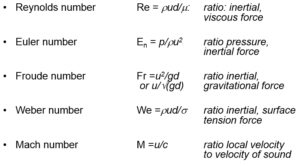# Dimensionless Numbers Used In Fluid Mechanics

Sharing is Caring :)-

Dimension less numbers have important role in fluid mechanics. With the help of these numbers we can easily predict the flow behavior of fluid. When any mass is in motion inertia force always exists. These dimension less numbers are formed by considering the ratio of inertia force to any one of the force from viscous force, gravity force, pressure force, surface tension force and elastic force. Each ratio gives a different dimensionless number used in fluid mechanics. Following are some dimensionless numbers used in fluid mechanics.1. Reynolds number
2. Froude’s number
3. Euler’s number
4. Weber number
5. Mach number

# Dimension Less Numbers:

### Reynolds Number:

It is named on British engineer Osborne Reynolds (1842-1912). Reynolds number is defined as the ratio of inertial force to viscous force.

Significance: Reynolds number signifies the relative predominance between inertia and viscous forces in fluid flow i.e. larger the Reynolds number greater will be the contribution of inertia effect where as in case of smaller Reynolds number greater will be the magnitude of viscous forces.

Reynolds number is always important in free surfaces as well as without free surfaces. It is only neglected in flow regions away from the high velocity gradients. It is applicable in case of incompressible flow of fluids in closed pipe, submarines completely under water, motion of air planes, jets, fluid flow involving viscous and inertial forces, pipe flow and fully submerged bodies.

### Froude’s number:

It is named on a British naval architect William Froude (1810-1887). He develops a ship model on towing tank concept and proposed similarity rules for free surface flows. Froude’s number is the ratio of inertial force to gravity force.

Significance: Its significance mainly in ship resistance, surface waves, spillway, notches and open channels. Froude’s number is dominant in free surfaces only. It has no significance if there are no free surfaces. It can also be defined as the ratio of mean velocity to the velocity of small waves in fluids.

### Euler’s number:

It is named on Leonhard Euler (1707-1783). Euler’s number is the ratio of inertial force to the pressure force.

Significance: Euler’s number has significance in cavitation i.e. when pressure drops  low enough  to vapour pressure but it is less important unless the pressure drops low enough to vapour pressure cause cavitation in liquids

### Weber number:

It is named on Moritz Weber (1871-1951). He developed the law of similitude. Weber number comes after Reynolds and Froude’s. Weber number is the ratio of inertial force to the surface tension force.

Significance: Weber number is significant only if it is less than unity which occurs only when the liquid surface curvature is very less as compare to depth of the liquid where surface tension force predominates for example droplets, capillarity flows, flow over weirs involving very low head and very thin sheets of liquid flowing over surface.

### Mach number:

It is named after Ernst Mach an Austrian physicist (1838-1916). Mach number is defined as the ratio of inertial force to the elastic force.

Significance:  Mach number has significance in compressible flow of fluids. It has strong effect if its value is greater than 0.3 i.e. velocity higher than the speed of sound. It has application in aerodynamic testing at high velocities. Mach number is also applied in case of unsteady flow like hammer blow.